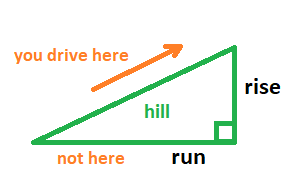Tutoring math, you encounter grade. The tutor discusses its definition and why it might be surprising.Grade is defined as 100%*(vertical/horizontal). In the above diagram, it would be as follows:

By itself, rise/run is called slope.

Therefore,

What follows is a distinction that, to me, is important and interesting:

rise/distance traveled

since, of course, you can’t drive along the horizontal course of a hill; rather, you can only drive on its surface.

At level, grade and (rise/distance traveled) are both zero. They remain virtually the same even at 20% grade, when (rise/distance traveled) is 19.6%. As the grade increases, however, they differ dramatically: at 100% grade, (rise/distance traveled) is 70.7%.

My interest in the difference between grade and (rise/distance traveled) is philosophical: why base a value on an indirect measurement (horizontal distance), when a direct measurement (distance traveled) is available?

In math, we use slope, of course; however, it’s usually in a context where actual measurements aren’t used. Rather, it’s just on paper.

Source:

engineeringtoolbox.com

connect.ubc.ca

Jack of Oracle Tutoring by Jack and Diane, Campbell River, BC.